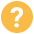Evaluating bids

# Derive Center of Gravity (Cog) to be in the line of symmetry of robotic arm used on drones (Matlab) (Price is negotiable*

Published on the June 21, 2020 in Engineering & Manufacturing

### USD 250 - 500NOTE: I am willing to negotiate the price, but, I would appreciate if there are no changes to the price after negotiating and no cancellation of the project after confirmation. Thank you for understanding. :))

Description:

Hi,

I have a MATLAB code that gives the output of the 5 bar parallel link robot.

This robot arm will be attached to the drone and a slider mechanism is to be included.

- For this case, with the slider mechanism, the COG has to always be on the line of symmetry as the robot arm slides.
- This would then bring effect about the end-effector position (described below).
- The design is such that it works on planar coordinates (XY plane).

The robot arm has to be such that it can move fast.

- The task which I am in need is to get a method to get the cog to be in the line of symmetry even if the slider moves with the motorized joints moving at a relatively fast rate (similar to the dexterous robots) and to be applied on matlab with the necessary calculations.
- This could require the use of Jacobian Matrix, derivations of equations of COG, and other steps. I have the pdf copy of the steps/calculations required and the MATLAB code for the Jacobian matrix calculations, but I think this approach might not work or could be improved.

- I would require a COG trajectory plot

Also, I would require the counter-balancing mechanism, probably a rotating mass attached to compensate for the angular momentum created by the movement of the robotic arm system. For this force graphs would be required.
A video of the counter balancing mechanism that is referred to is attached here:
- https://youtu.be/Bk1QmccUaZY

1.Keeping cog in line of symmetry as the slider mechanism moves with trajectory plots of the cog (matlab) and matlab simulation
2.

The end effector position: refers to controlling the position of the slider and the input joints at the base to achieve the desired position. So, if the end effector is in position x0, y0 and you want to reach position x1, y1, what are the values of the slider and thethas to achieve that while keeping the CoG in the centre.
3. Counterbalancing mechanism for the robot arm (rotating mass) and force graphs
4. Require detailed report for the steps and calculations derived

I have added a document that is similar to that of my requirements here, but the methods required may vary, and the MATLAB codes necessary.

NOTEs on the documents attached:
1. WithoutPatch_COMFixed: The MATLAB code for the 5 bar parallel links
2. BackgroundUnderstanding: A paper that has been attempted by using the Jacobian matrix method, but in this case for a robotic arm with 2 links.

3. 5 link robot (2): An attempt of using the Jacobian matrix method described in BackgroundUnderstanding
4. Screenshot: An simple illustration of how the system will look like
5. Task Objectives: A document stating the precise objectives of the task.

Project overview

- Robotics - Mathematics - MATLAB Skills - Experience working with robot arms

Category Engineering & Manufacturing
Subcategory Other
Project size Medium
Is this a project or a position? Project
Required availability As needed